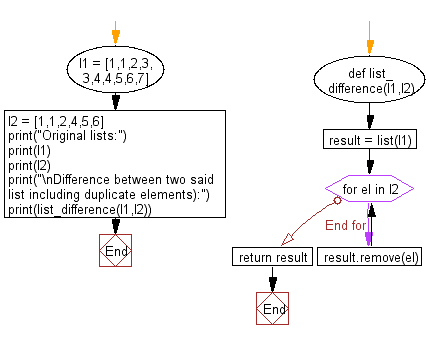﻿ Python: Find the difference between two list including duplicate elements - w3resource# Python: Find the difference between two list including duplicate elements

## Python List: Exercise - 134 with Solution

Write a Python program to find the difference between two list including duplicate elements.

Sample Solution:

Python Code:

``````def list_difference(l1,l2):
result = list(l1)
for el in l2:
result.remove(el)
return result
l1 = [1,1,2,3,3,4,4,5,6,7]
l2 = [1,1,2,4,5,6]

print("Original lists:")
print(l1)
print(l2)
print("\nDifference between two said list including duplicate elements):")
print(list_difference(l1,l2))
```
```

Sample Output:

```Original lists:
[1, 1, 2, 3, 3, 4, 4, 5, 6, 7]
[1, 1, 2, 4, 5, 6]

Difference between two said list including duplicate elements):
[3, 3, 4, 7]
```

Flowchart:## Visualize Python code execution:

The following tool visualize what the computer is doing step-by-step as it executes the said program:

Python Code Editor:

Have another way to solve this solution? Contribute your code (and comments) through Disqus.

What is the difficulty level of this exercise?

Test your Python skills with w3resource's quiz

﻿

## Python: Tips of the Day

Floor Division:

When we speak of division we normally mean (/) float division operator, this will give a precise result in float format with decimals.

For a rounded integer result there is (//) floor division operator in Python. Floor division will only give integer results that are round numbers.

```print(1000 // 300)
print(1000 / 300)```

Output:

```3
3.3333333333333335```